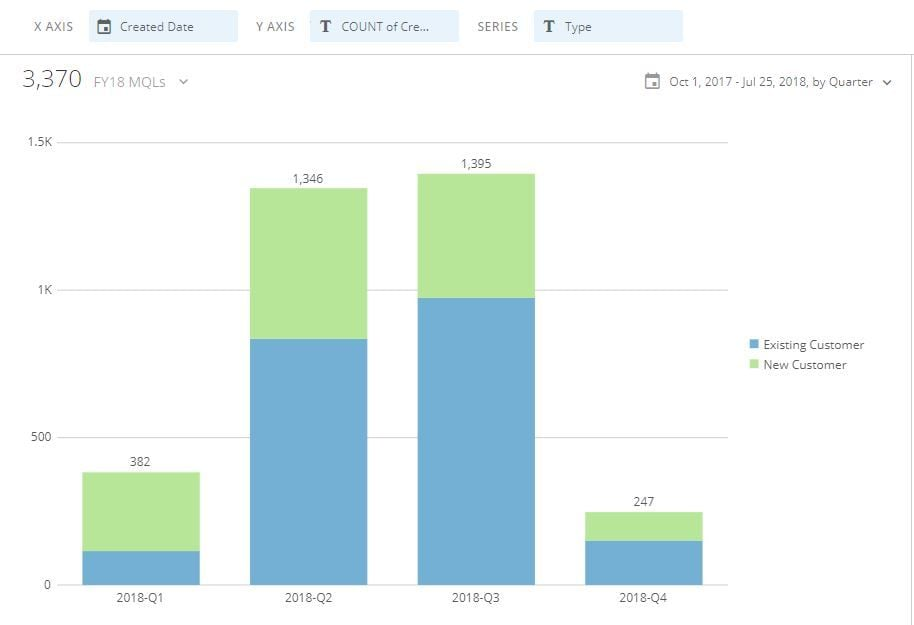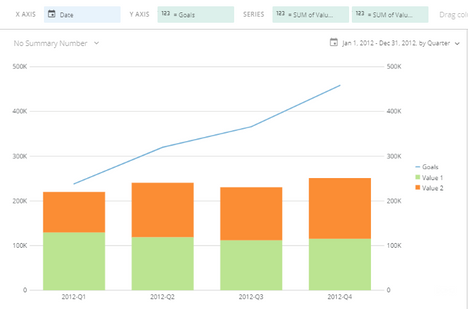# Setting Goals for each Quarter in Bar Chart

I am trying to set goals for each individual quarter. My data is currently represented as a stacked bar chart and would like to make the goals a line. My series is calculating the count of each type of lead for each fiscal quarter. I am having trouble with my beastmode calculation setting the goal value of leads for each fiscal quarter. This is how my calculation is currently and I am getting a syntax error:

CASE
WHEN `Created Date` = '2018-Q1' THEN Count(`Type`='518')
WHEN `Created Date` = '2018-Q2' THEN COUNT(`Type`= '1224')
WHEN `Created Date` = '2018-Q3' THEN COUNT (`Type`='1373')
WHEN `Created Date` = '2018-Q4' THEN COUNT (`Type`= '1778')
ENDI would like the goals for each quarter to be placed on a line on top of the bar graph.

Anyone have a solution?

• Looks like in your beast mode you're trying to either override the count function or set the value of the count with = syntax.  Case statements won't work that way.

It should be more like

CASE
WHEN `Created Date` = '2018-Q1' THEN 518
WHEN `Created Date` = '2018-Q2' THEN 1224
WHEN `Created Date` = '2018-Q3' THEN 1373
WHEN `Created Date` = '2018-Q4' THEN 1778
END

There may still be an issue with the way that date value is stored and compared here.

Backing up, your approach of individualized goals with a beast mode is good.  Here's how I've solved this question in the past.This is a "line and stacked bar" chart, with each goal or value as an independent beast mode.

Goal beast mode, similar to yours:

case
when QUARTER(date) = 1 then 1000
when QUARTER(date) = 2 then 1250
when QUARTER(date) = 3 then 1500
when QUARTER(date) = 4 then 1750
end

Value 1 beast mode:

case when `Group` = 'Group 1' then `Value1` end

Value 2 beast mode:

case when `Group` = 'Group 2' then `Value1` end

Make sure to have the goal line first.  Otherwise it shows up as a segment on the stacked bar.